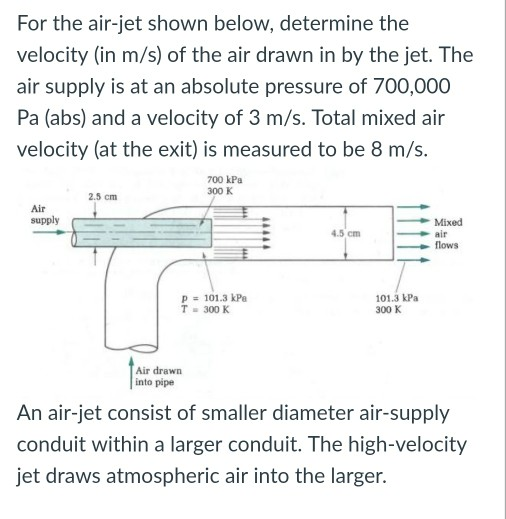# For the air-jet shown below, determine the velocity (in m/s) of the air drawn in by...

###### Question:For the air-jet shown below, determine the velocity (in m/s) of the air drawn in by the jet. The air supply is at an absolute pressure of 700,000 Pa (abs) and a velocity of 3 m/s. Total mixed air velocity (at the exit) is measured to be 8 m/s. 700 kPa 300 K 2.5 cm Air supply Mixed air flows 45 cm - p 101.3 kPa T-300 K 101.3 kPa 300 K Air drawn into pipe An air-jet consist of smaller diameter air-supply conduit within a larger conduit. The high-velocity jet draws atmospheric air into the larger.

#### Similar Solved Questions

##### Express the confidence interval 422.2 149.1 in the form of a trilinear inequality < < Express...
Express the confidence interval 422.2 149.1 in the form of a trilinear inequality < < Express the confidence interval 576.1 <u < 702.9 in the form of ME. IME = Express the confidence interval (66.3, 165.5) in the form of ö ME. + ME=...